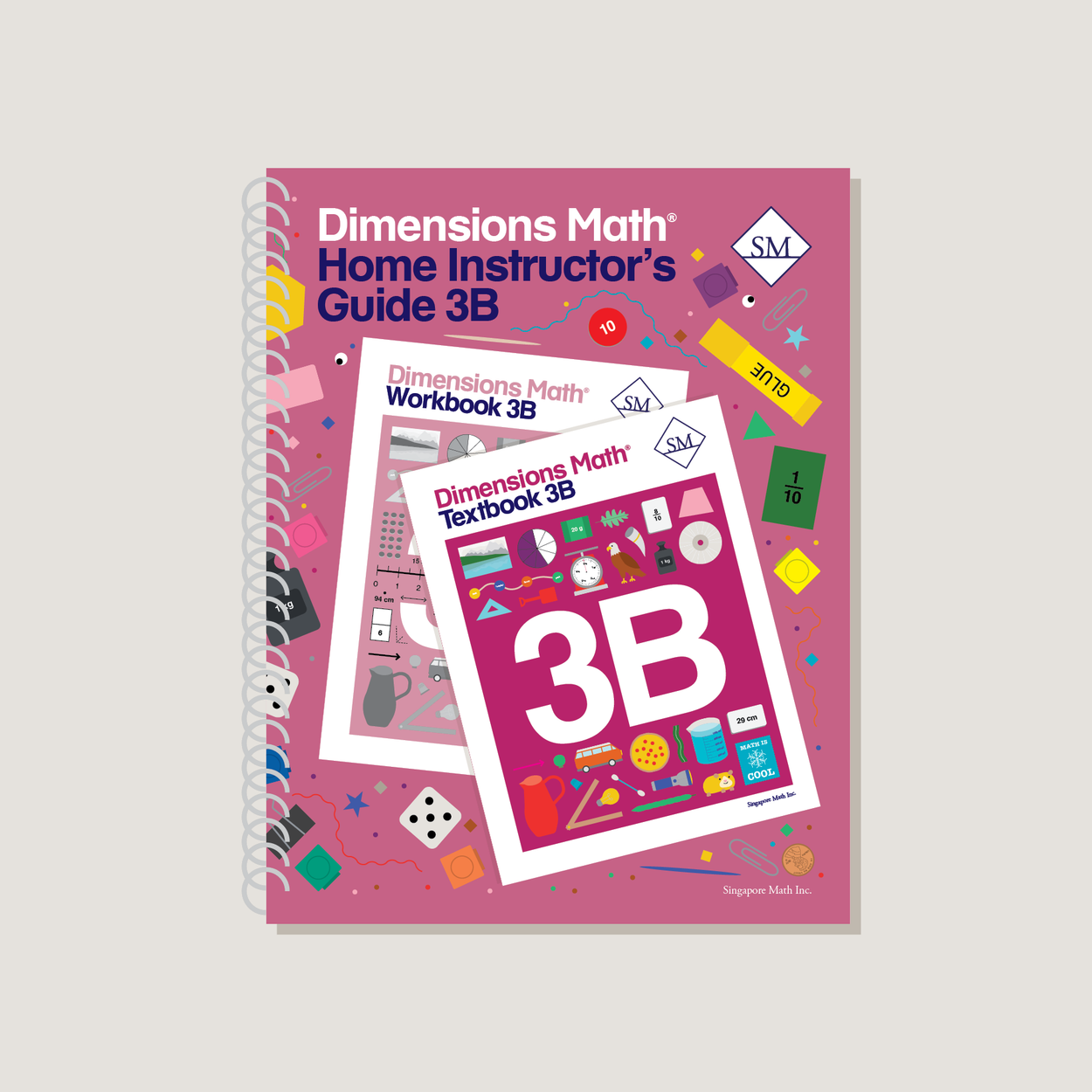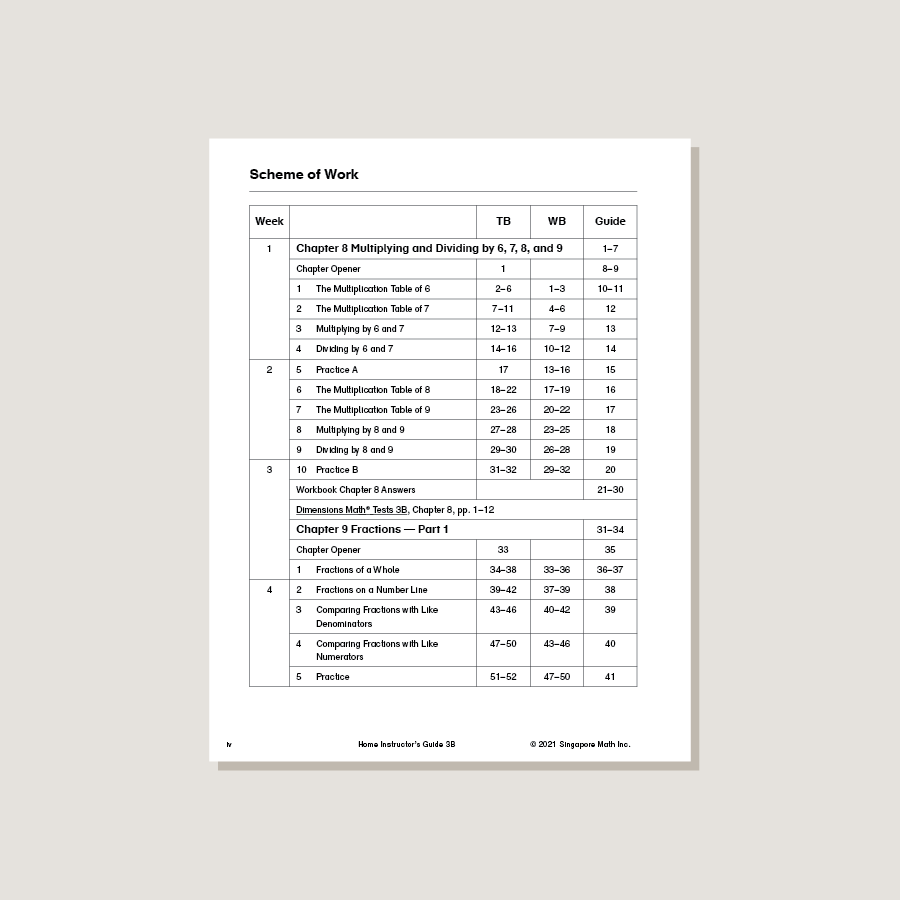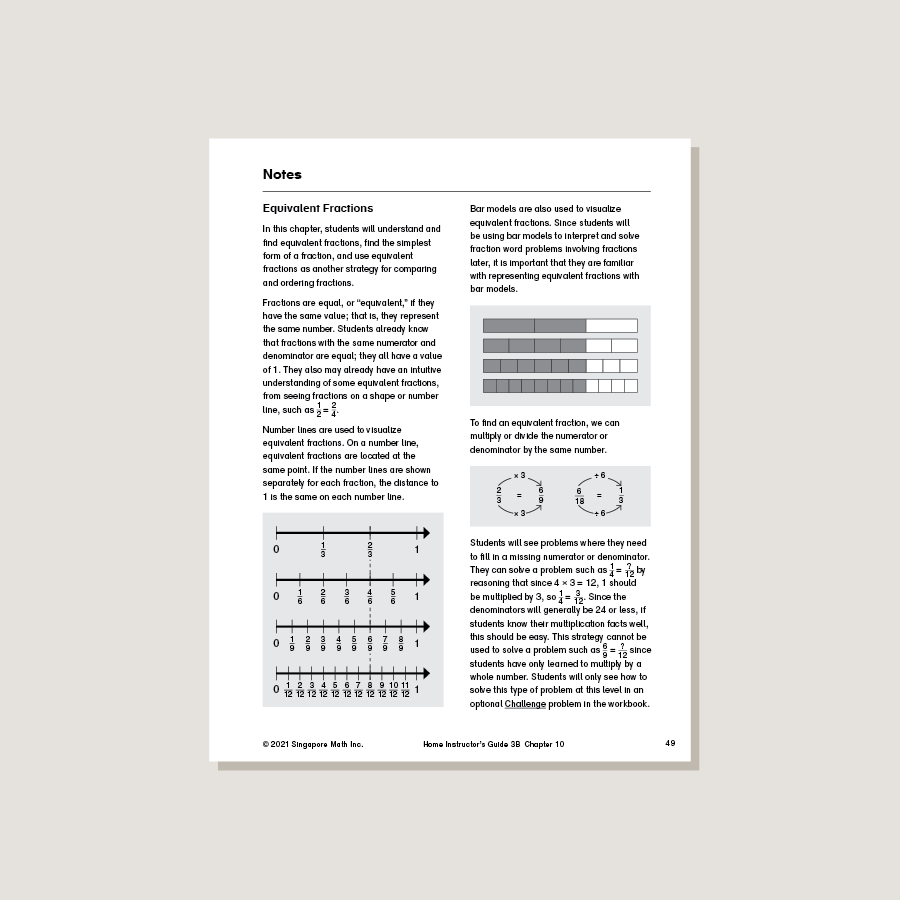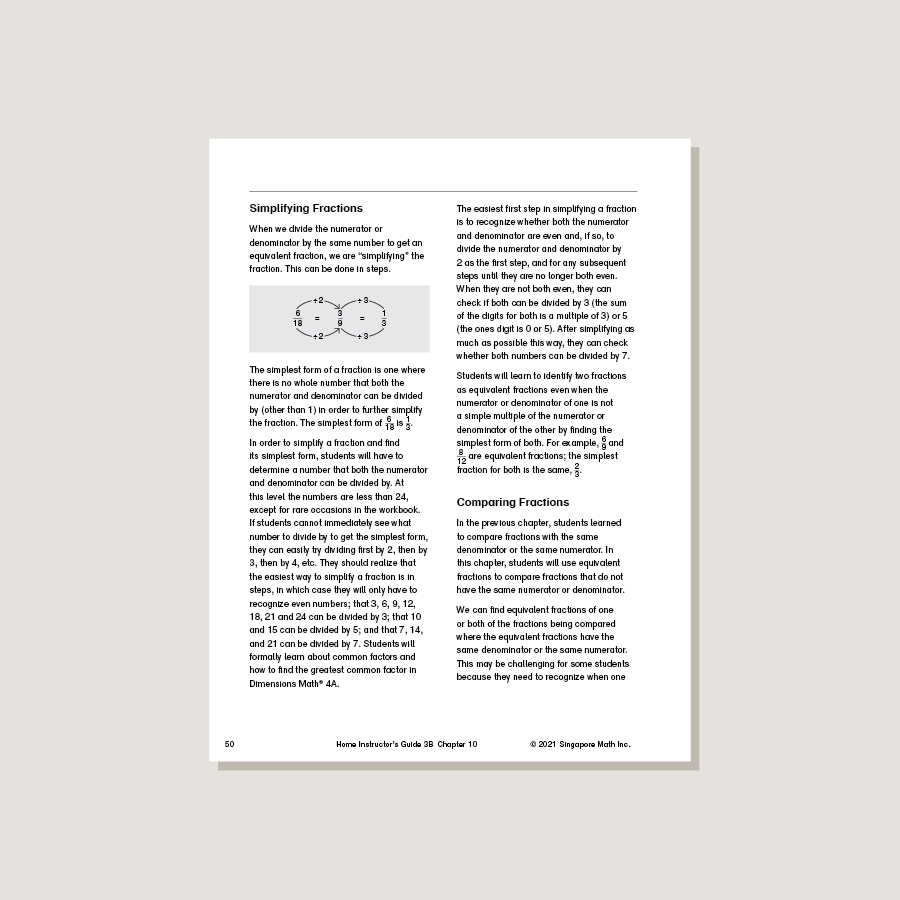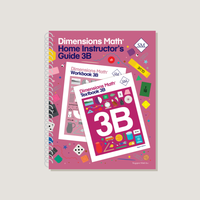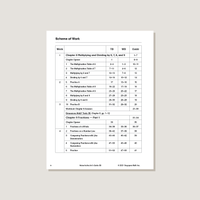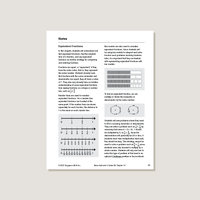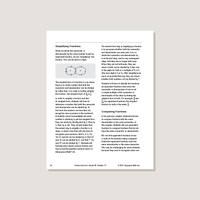# Dimensions Math Home Instructor's Guide 3B

\$21.40

Dimensions Math Home Instructor’s Guides are an essential resource for teaching Dimensions Math in a home or one-on-one setting. The purpose of the Guides is to help educators understand concepts in the Dimensions Math curriculum and teach as effectively as possible. They include extensive background notes, lesson suggestions, tips, and activities. Home Instructor’s Guides include answer keys for corresponding Dimensions Math Textbooks and Workbooks.

Note: Soft cover, spiral bound.

SKU: DMHIG3B

ISBN: 9781947226630

Pagecount: 208

Dimensions: 8.5 x 11 x 0.5 in

Sample Pages

Chapter 8: Multiplying and Dividing with 6, 7, 8, and 9
Lesson 1: The Multiplication Table of 6
Lesson 2: The Multiplication Table of 7
Lesson 3: Multiplying by 6 and 7
Lesson 4: Dividing by 6 and 7
Lesson 5: Practice A
Lesson 6: The Multiplication Table of 8
Lesson 7: The Multiplication Table of 9
Lesson 8: Multiplying by 8 and 9
Lesson 9: Dividing by 8 and 9
Lesson 10: Practice B

Chapter 9: Fractions — Part 1
Lesson 1: Fractions of a Whole
Lesson 2: Fractions on a Number Line
Lesson 3: Comparing Fractions with Like Denominators
Lesson 4: Comparing Fractions with Like Numerators Lesson 5: Practice

Chapter 10: Fractions — Part 2
Lesson 1: Equivalent Fractions
Lesson 2: Finding Equivalent Fractions
Lesson 3: Simplifying Fractions
Lesson 4: Comparing Fractions — Part 1
Lesson 5: Comparing Fractions — Part 2
Lesson 6: Practice A
Lesson 7: Adding and Subtracting Fractions — Part 1
Lesson 8: Adding and Subtracting Fractions — Part 2
Lesson 9: Practice B

Chapter 11: Measurement
Lesson 1: Meters and Centimeters
Lesson 2: Subtracting from Meters
Lesson 3: Kilometers
Lesson 4: Subtracting from Kilometers
Lesson 5: Liters and Milliliters
Lesson 6: Kilograms and Grams
Lesson 7: Word Problems
Lesson 8: Practice
Review 3

Chapter 12: Geometry
Lesson 1: Circles
Lesson 2: Angles
Lesson 3: Right Angles
Lesson 4: Triangles
Lesson 5: Properties of Triangles
Lesson 7: Using a Compass
Lesson 8: Practice

Chapter 13: Area and Perimeter
Lesson 1: Area
Lesson 2: Units of Area
Lesson 3: Area of Rectangles
Lesson 4: Area of Composite Figures
Lesson 5: Practice A
Lesson 6: Perimeter
Lesson 7: Perimeter of Rectangles
Lesson 8: Area and Perimeter
Lesson 9: Practice B

Chapter 14: Time
Lesson 1: Units of Time
Lesson 2: Calculating Time — Part 1
Lesson 3: Practice A
Lesson 4: Calculating Time — Part 2
Lesson 5: Calculating Time — Part 3
Lesson 6: Calculating Time — Part 4
Lesson 7: Practice B

Chapter 15: Money
Lesson 1: Dollars and Cents
Lesson 2: Making \$10
Lesson 4: Subtracting Money
Lesson 5: Word Problems
Lesson 6: PracticeReview 4
Review 5

A & B Books: Our programs divide the school year into two semesters. “A” level books are for the first half of the school year. “B” level books are for the second half of the school year. You need both “A” and “B” material for a complete school year.

Required Components: Textbooks, Workbooks, and Guides (either Home Instructor’s Guides or Teacher’s Guides) are all necessary components. These three elements each serve a unique function and work together to build math mastery.

Printouts: Dimensions Math PK-5 Resources

Recommended Manipulatives: Dimensions Math Grades PK-5 Recommended Manipulatives